Next: 5.3.5 Direction Vector Sampling Up: 5.3 Generation of Random Previous: 5.3.3 Direction Vector Calculation

## 5.3.4 Cosine Distribution

The most frequently used distribution is the cosine distribution, which is required to describe the arrival angles of neutral particles (2.5) or diffusive reemission (2.18). The cosine distribution can be seen as a special case of the power cosine distribution (2.6) with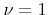or as a special case of the coned cosine distribution with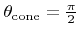. As shown in the next section, sampling a cosine distribution using Algorithm 5.2 is faster than using Algorithm 5.3 for the coned cosine distribution. Therefore, the power cosine distribution withis the common technique for sampling a cosine distribution . However, the polar angle is usually not of interest. It is just an intermediate result passed to Algorithm 5.4 to generate a unit vector as needed for ray tracing.

In the following discussion an alternative method is proposed, which samples a unit vector obeying the cosine distribution directly, without the need of an additional rotation as described in Section 5.3.3. The method uses the fact that the sum of the given direction vector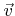and a unit random vector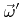, which is uniformly distributed over a sphere, follows a cosine distribution. As illustrated in Figure 5.6, the area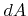seen from the origin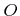in a direction with polar angle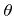within an infinitesimal solid angle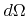is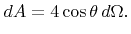(5.52)

As a consequence, the desired random vector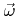can be obtained from a spherically distributed unit vectorby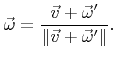(5.53)

For the spherical distribution numerous methods have been proposed in the past. So far the most efficient way to pick a point on the unit sphere is described in [61,75,107] and is listed in Algorithm 5.5. Standard numeric libraries such as the GNU scientific library already provide ready implementations for that algorithm . Therefore, a very simple and short vector sampling algorithm for the cosine distribution is given by Algorithm 5.6.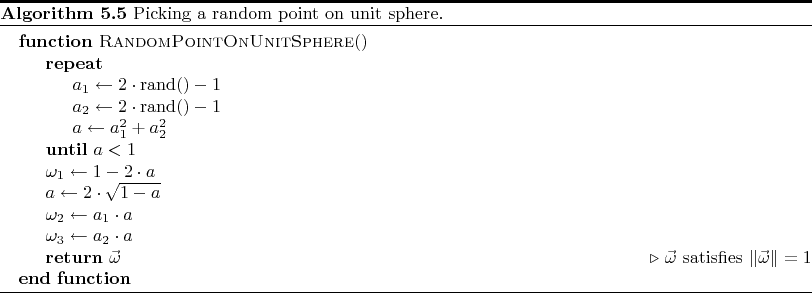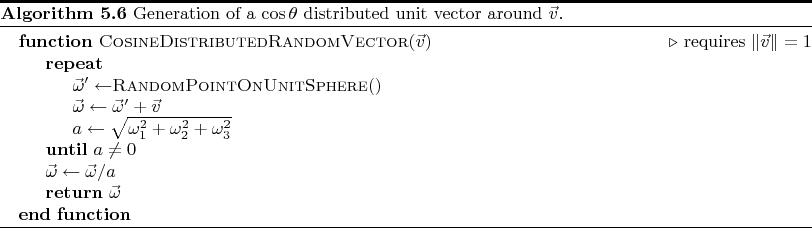Next: 5.3.5 Direction Vector Sampling Up: 5.3 Generation of Random Previous: 5.3.3 Direction Vector Calculation

Otmar Ertl: Numerical Methods for Topography Simulation